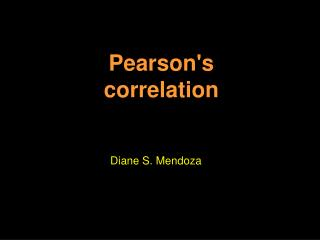DownloadDownload PresentationPearson's correlation

# Pearson's correlation

Télécharger la présentation## Pearson's correlation

- - - - - - - - - - - - - - - - - - - - - - - - - - - E N D - - - - - - - - - - - - - - - - - - - - - - - - - - -
##### Presentation Transcript

1. Pearson's correlation Diane S. Mendoza

2. It is named after Karl Pearson who developed the correlational method to do agricultural research. • designated by the Greek letter rho (ρ) • The product moment part of the name comes from the way in which it is calculated, by summing up the products of the deviations of the scores from the mean. • A correlation is a number between -1 and +1 that measures the degree of association between two variables (call them X and Y). • A positive value for the correlation implies a positive association • A negative value for the correlation implies a negative or inverse association

3. The formula for the Pearson correlation Suppose we have two variables X and Y, with means XBAR and YBAR respectively and standard deviations SX and SY respectively. The correlation is computed as as the sum of the product of the Z-scores for the two variables divided by the number of scores.

4. If we substitute the formulas for the Z-scores into this formula we get the following formula for the Pearson Product Moment Correlation Coefficient, which we will use as a definitional formula. The numerator of this formula says that we sum up the products of the deviations of a subject's X score from the mean of the Xs and the deviation of the subject's Y score from the mean of the Ys. This summation of the product of the deviation scores is divided by the number of subjects times the standard deviation of the X variable times the standard deviation of the Y variable

5. When will a correlation be positive? • Suppose that an X value was above average, and that the associated Y value was also above average. Then the product would be the product of two positive numbers which would be positive. • If the X value and the Y value were both below average, then the product above would be of two negative numbers, which would also be positive. • Therefore, a positive correlation is evidence of a general tendency that large values of X are associated with large values of Y and small values of X are associated with small values of Y.

6. When will a correlation be negative? • Suppose that an X value was above average, and that the associated Y value was instead below average. Then the product would be the product of a positive and a negative number which would make the product negative. • If the X value was below average and the Y value was above average, then the product above would be also be negative. • Therefore, a negative correlation is evidence of a general tendency that large values of X are associated with small values of Y and small values of X are associated with large values of Y.

7. Interpretation of the correlation coefficient The correlation coefficient measures the strength of a linear relationship between two variables. The correlation coefficient is always between -1 and +1. The closer the correlation is to +/-1, the closer to a perfect linear relationship. Here is to interpret correlations. -1.0 to -0.7 strong negative association. -0.7 to -0.3 weak negative association. -0.3 to +0.3 little or no association. +0.3 to +0.7 weak positive association. +0.7 to +1.0 strong positive association.

8. Let's calculate the correlation between Reading (X) and Spelling (Y) for the 10 students. There is a fair amount of calculation required as you can see from the table below. First we have to sum up the X values (55) and then divide this number by the number of subjects (10) to find the mean for the X values (5.5). Then we have to do the same thing with the Y values to find their mean (10.3).

9. Formula : We then calculate : The correlation we obtained was -.36, showing us that there is a small negative correlation between reading and spelling. The correlation coefficient is a number that can range from -1 (perfect negative correlation) through 0 (no correlation) to 1 (perfect positive correlation).

10. The computational formula for the Pearsonian r is • By looking at the formula we can see that we need the following items to calculate r using the raw score formula: • The number of subjects, N • The sum of each subjects X score times the Y score, summation XY • The sum of the X scores, summation X • The sum of the Y scores, summation Y • The sum of the squared X scores, summation X squared • The sum of the squared Y scores, summation Y squared

11. In we plug each of these sums into the raw score formula we can calculate the correlation coefficient: We can see that we got the same answer for the correlation coefficient (-.36) with the raw score formula as we did with the definitional formula.

12. GRACIAS!